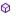Press on the image to return to the main documentation page.

# ExpressionEvaluator

### Written by Andrew Graham

This library includes an Evaluator object that can evaluate arithmetic expressions.

Evaluator

### Evaluator

This class contains an arithmetic expression evaluator.
It supports the arithmetic sub-set of the full Basic4android language.

Operators in precedence order are

^ : exponent
* / mod : multiply, divide and modulus, % is also accepted for mod
+ - : add and subtract

Functions supported are

COS(r), SIN(r), TAN(r), ACOS(n), ASIN(n), ATAN(n) : radians
COSD(d), SINDd), TANDd), ACOSD(n), ASIND(n), ATAND(n) : degrees
LN(n) base e, LOG(n) base 10, LOGARITHM(n, base) any base
SQRT(n), FLOOR(n), ABS(n), MAX(n1, n2), MIN(n1, n2), POWER(n, exp), ROUND(n)

Constants recognised are
CPI : Pi
CE : e

Twenty-six case-insensitive variables are supported named from "A" to "Z" and must be intialised with the required value before being used in an evaluation.
Although the actual variable names are a single letter longer names are accepted but only the initial letter is used to identify the variable.
Variable values are maintained across evaluations.

None

#### Members:Error As String [read only]ErrorFlag As Boolean [read only]Evaluate (expression As String) As DoubleEvaluateAndSave (expression As String, var As String) As DoubleGetGlobal (var As String) As DoubleInitializeSetGlobal (var As String, value As Double)Version As Double [read only]

#### Members description:

#####ErrorAsString [read only]
Returns the error if any of the last evaluation otherwise returns an empty string.
If the error is a NullPointerException or a StringIndexException then the evaluated expression was ill-formed.
#####ErrorFlagAsBoolean [read only]
Returns True if an error occured in the last call to Evaluate otherwise returns False.
#####Evaluate (expressionAsString) AsDouble
Evaluate the supplied expression and return the result.
If an error occurs zero is returned and the Error property will contain the reason.
#####EvaluateAndSave (expressionAsString, varAsString) AsDouble
Evaluate the supplied expression and return the result also saving it in the specified variable.
#####GetGlobal (varAsString) AsDouble
Returns the value of the variable specified.
Twenty-six case-insensitive variables are supported named from "A" to "Z" longer names are accepted but only the initial letter is used.
#####Initialize
Initialise the Evaluator.
#####SetGlobal (varAsString, valueAsDouble)
Sets the contents of the variable named var to value.
Twenty-six case-insensitive variables are supported named from "A" to "Z" longer names are accepted but only the initial letter is used.
#####VersionAsDouble [read only]
Returns the version number of the library.
Top